Courses

# Transformation of Stress & Strain - 1 Civil Engineering (CE) Notes | EduRev

## Civil Engineering (CE) : Transformation of Stress & Strain - 1 Civil Engineering (CE) Notes | EduRev

The document Transformation of Stress & Strain - 1 Civil Engineering (CE) Notes | EduRev is a part of the Civil Engineering (CE) Course Solid Mechanics.
All you need of Civil Engineering (CE) at this link: Civil Engineering (CE)

Principal Stress and Principal Strain

Different states of stress

Depending upon the state of stress at a point, we can classify it as uniaxial(1D), biaxial(2D) and triaxial(3D) stress.

1. One dimensional stress(Uniaxial)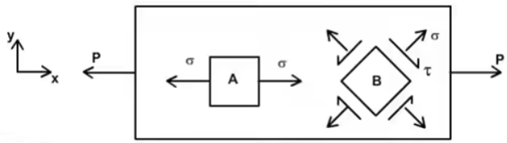Consider a bar under a tensile load P acting along its axis as shown in the figure. Take an element A which has its sides parallel to the surfaces of the bar. It is clear that the element has only normal stress along only one direction, i.e., x-axis and all other stresses are zero. Hence it is said to be under uni-axial stress state. Now consider another element B in the same bar, which has its slides inclined to the surfaces of the bar. Though the element has normal and shear stresses on each face, it can be transformed into a uni-axial stress state like element A by transformation of stresses
Now consider another element B in the same bar, which has its slides inclined to the surfaces of the bar. Though the element has normal and shear stresses on each face, it can be transformed into a uni-axial stress state like element A by transformation of stresses.
2. Two-dimensional stress (Plane stress)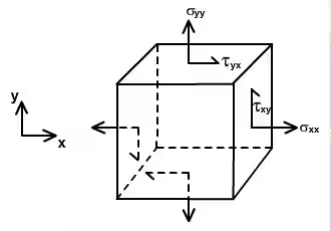When the cubic element is free from any of the stresses on its two parallel surfaces and the stress components in the element can not be reduced to a uni-axial stress by transformation, then, the element is said to be in two-dimensional stress/plane stress state. Thin plates under mid plane loads and the free surface of structural elements may experience plane stresses as shown below.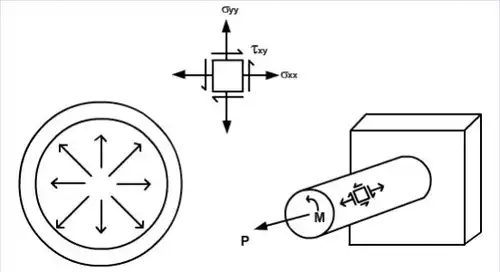Transformation of plane stress

Though the state of stress at a point in a stressed body remains the same, the normal and shear stress components vary as the orientation of plane through that point changes. Under complex loading, a structural member may experience larger stresses on inclined planes then on the cross section. The knowledge of maximum normal and shear stresses and their plane's orientation assumes significance from failure point of view. Hence, it is important to know how to transform the stress components from one set of coordinate axes to another set of co-ordinates axes that will contain the stresses of interest.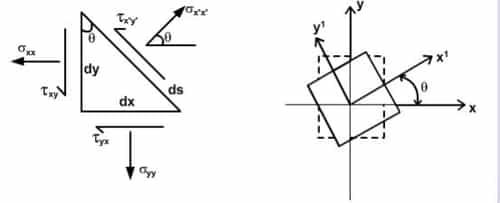Consider a prismatic element with sides dx, dy and ds with their faces perpendicular to y, x and x' axes respectively. Thickness of the element is t. σ and τ = are the normal and shear stresses acting on a plane inclined at an angle θ measured counter clockwise from x plane.

Under equilibrium, ∑FX' = 0 & ∑FY' = 0 and on simplifying we get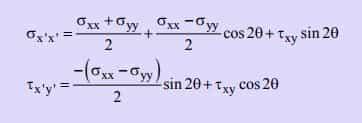Replacing θ by θ + 90º , in expression of equation, we get the normal stress along y' direction.
σy'y' = ((σxx + σyy) / 2)-((σxx σyy) / 2)cos2θ - τxy sin2θ

Above Equations are the transformation equations for plane stress using which the stress components on any plane passing through the point can be determined. Notice here that, σxx + σyy = σx'x' + σy'y'

Invariably, the sum of the normal stresses on any two mutually perpendicular planes at a point has the same value. This sum is a function of the stress at that point and not on the orientation of axes. Hence, this quantity is called stress invariant at that a point.

1. Principal stresses and maximum shear stress
From transformation equations, it is clear that the normal and shear stresses vary continuously with the orientation of planes through the point. Among those varying stresses, finding the maximum and minimum values and the corresponding planes are important from the design considerations. By taking the derivative of σx 'x ' in equation  with respect to  θ and equating it to zero, we get
tan2θP = 2τxy/(σxx - σyy)
Here, θp has two values θp1, and θp2 that differ by 90o with one value between 0o and 90o and the other between 90o and 180o . These two values define the principal planes that contain maximum and minimum stresses.
These two values define the principal planes that contain maximum and minimum stresses. Substituting these two θp values in first equations, the maximum and minimum stresses, also called as principal stresses, are obtained.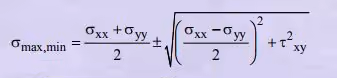The plus and minus signs in the second term of the above equation, indicate the algebraically larger and smaller principal stresses, i.e. maximum and minimum principal stresses.
In the equation , if τx'y is taken as zero, then the resulting equation is same as equation of tan2θP.
Thus, the following important observation pertained to principal planes is made.
2. The shear stresses are zero on the principal planes
To get the maximum value of the shear stress, the derivative of τx'y'  in equation with respect to θ is equated to zero and by solving we get
tan2θs = -(σxx - σyy) / 2τxy
Hence, θs has two values, θs1 and θs2 that differ by 90o with one value between 0o and 90o and the other between 90o and 180o .
Hence, the maximum shear stresses that occur on those two mutually perpendicular planes are equal in algebraic value and are different only in sign due to its complementary property.
Comparing above equations ,
tan2θP = -(1 / tan2θs)
It is understood from above equation that the tangent of the angles 2θp and 2θs are negative reciprocals of each other and hence, they are separated by 90o . Hence, we can conclude that θp and θs differ by 45o, i.e., the maximum shear stress planes can be obtained by rotating the principal plane by 45o in either direction.
The principal planes do not contain any shear stress on them, but the maximum shear stress planes may or may not contain normal stresses as the case may be. Maximum shear stress value is found out by substituting θs values in the first equation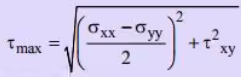Another expression for τmax is obtained from the principal stresses,
τmax = (σmax - σmin) / 2

Example: σx = -46 MPa σy = 12MPa
τxy = τyx = -19MPa
determine the stresses for θ = -150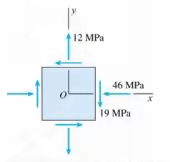Solution: (σ+ σy) / 2 = -17MPa, (σ- σy) / 2 = -29MPa
sin 2θ = sin(-300) = -0.5, cos2θ = cos(-300) = 0.866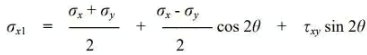-17 + (-29)0.866 + (-19)(-0.5) = -32.6MPa
τxlyl = -((σ- σy) / 2)sin2θ + τxycos2θ
= -(-29)(-0.5) + (-19)0.866
= -31MPa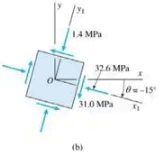σxl + σyl = σx + σy
σyl = σxl + σy - σx
= -46 + 12 - (-32.6) = -1.4MPa

Mohr's Circle for Plane Stress

Mohr’s circle is the locus of points representing magnitude of normal and shear stress at various plane in a given stress element. Graphically, variation of normal stress and shear stress are studied with the help of Mohr's circle.
σ1, σ2 are Principal Stress  then normal and shear stress on lane  which is inclined at angle ‘θ’ from major principal plane, then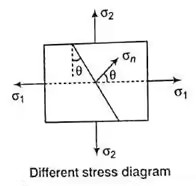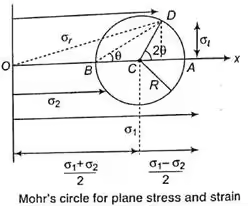Normal stress:
σn = ((σ1 + σ2) / 2) + ((σ1 - σ2)2)cos2θ

Shear stress:
τ = -((σ1 + σ2) / 2)sin2θ

General State of Stress at an Element

If  σxy are normal stress on vertical and horizontal plane respectively and this plane is  accompanied by shear stress  then normal stress and shear stress on plane, which is inclined at an angle θ from plane of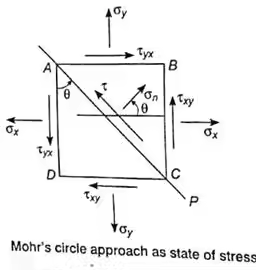then,
σθ = ((σ+ σy) / 2)+((σ- σy) / 2).cos2θ + τxy sin2θ
τθ = ((σ- σy) / 2) sin2θ - τxycos2θ
Let  be two normal stresses(both tensile) and  be shear stress then,

• Maximum and Minimum Principal Stresses are: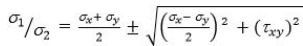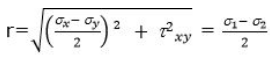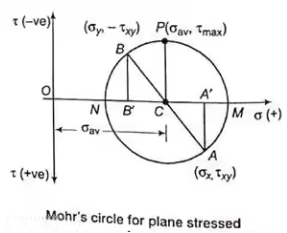Observations from Mohr's Circle

The following are the observations of Mohr's circle as

• At point M on circle σn is maximum and shear stress is zero.
∴ Maximum principal stress ≡ coordinate of M
• At point N on circle σn is minimum and shear stress τ is zero.
∴ minimum principal stress ≡ coordinate of N
• At point P on Circle τ is maximum.
Maximum shear stress ≡ ordinate of P(i.e. radius of circle)
Also, normal stress on plane of maximum shear stress
≡ abscissa of P((i.e., σn = σav = (σx + σy) / 2))
Where, σn ≡ Average stress
• Mohr's circle becomes zero at a point if radius of circle has the following consideration.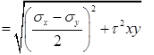• If σx = σy, then radius of Mohr's circle is zero and τxy = 0.
Offer running on EduRev: Apply code STAYHOME200 to get INR 200 off on our premium plan EduRev Infinity!

## Solid Mechanics

30 videos|27 docs|29 tests

,

,

,

,

,

,

,

,

,

,

,

,

,

,

,

,

,

,

,

,

,

;